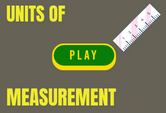# Measurement Units List Game Quiz Online

This page features a Measurement Units List Game Quiz Online. The units of measurement differ depending on what we are measuring. For example the units for measuring atmospheric pressure is different from that of measuring lengths. This interactive game will teach you units of measurement in a fun way. Great resource for classrooms and individual practice. Click and start learning. 5th, 6th, 7th, 8th and 9th grades.## SI Units and Measurements

The metric system is also known as the International System of Units, or SI. What does SI mean?

1. System International
2. Systemic International
3. Standard International
4. Système International

The metric system is based on the…

1. Powers of 10
2. Square roots of 10
3. Powers of 8
4. Powers of 4

How many grams are in a kilogram?

1. 10 g
2. 100 g
3. 1,000 g
4. 10,000 g

What is the SI unit for temperature?

1. Celsius (°C)
2. Fahrenheit (°F)
3. Kelvin (K)
4. Rankine (°R)

How many millimetres are in a hectometre?

1. 1,000 mm
2. 10,000 mm
3. 100,000 mm
4. 1,000,000 mm

The “mol” is the SI unit that measures the number of particles in a substance. What does mol mean?

1. Mole
2. Mohl
3. Mool
4. Moll

Convert 1 inch into its value in centimeters.

1. 1.27 cm
2. 2.54 cm
3. 12.7 cm
4. 25.4 cm

Which of these tools is used for measuring lengths?

1. Ruler
2. Meter stick
3. Tape measure
4. All of the above

Each of these items arranges the metric prefixes from largest to smallest, except for one. Which is it?

1. Tera, giga, mega
2. Kilo, deca, hecto
3. Deka, deci, centi
4. Milli, micro, nano

How many seconds are there in a day?

1. 36,000 s
2. 72,000 s
3. 86,400 s
4. 92,300 s

What is 33 cm in meters?

1. 0.033 m
2. 0.33 m
3. 3.3 m
4. 33 m

Which of these is a unit of area?

1. Kilometer
2. Centimeter
3. Cubic meter
4. Square meter

A liter is a unit measuring three-dimensional space, or volume. It is equivalent to…

1. 1,000,000 cubic millimeters
2. 1,000 cubic centimeters
3. 1 cubic decimeter
4. All of the above

Which SI unit below is not matched to its appropriate measuring tool?

1. Mass : Triple beam balance
2. Length : Meter stick
3. Time : Thermometer